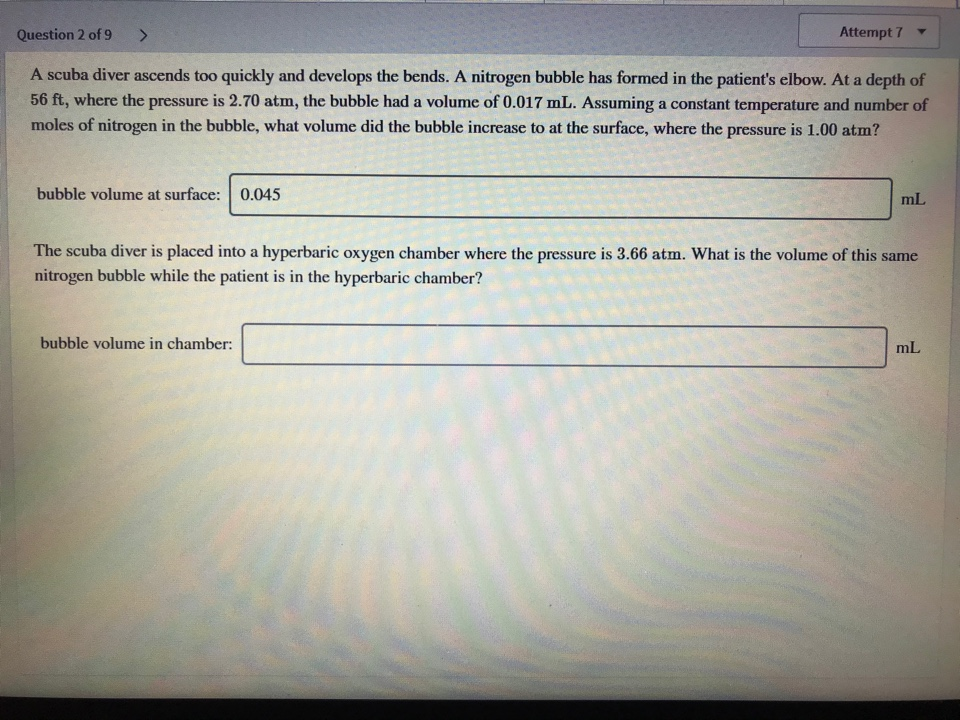# A scuba diver ascends too quickly and develops the bends. A nitrogen bubble has formed in the patient's elbow. At a depth of 56 ft, where the pressure is 2.70 atm, the bubble had a volume of 0.017 mL. Assuming a constant temperature and number of moles of nitrogen in the bubble, the bubble volume at the surface, where the presure is 1.00 atm is 0.045 mL. The scuba diver is placed into a hyperbaric oxygen chamber where the pressure is 3.66 atm. What is the volume of this same nitrogen bubble while the patient is in the hyperbaric chamber? bubble volume in chamber?